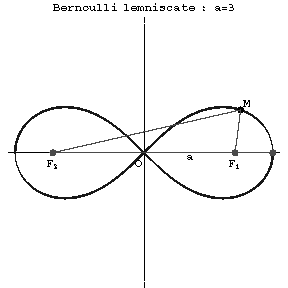Bernoulli lemniscate

A plane algebraic curve of order four, the equation of which in orthogonal Cartesian coordinates is:

$$(x^2+y^2)^2-2a^2(x^2-y^2)=0;$$

and in polar coordinates

$$\rho^2=2a^2\cos2\phi.$$

The Bernoulli lemniscate is symmetric about the coordinate origin (Fig.), which is a node with tangents $y=\pm x$ and the point of inflection.Figure: b015620a

The product of the distances of any point $M$ to the two given points $F_1(-a,0)$ and $F_2(a,0)$ is equal to the square of the distance between the points $F_1$ and $F_2$. The Bernoulli lemniscate is a special case of the Cassini ovals, the lemniscates, and the sinusoidal spirals (cf. Cassini oval; Sinusoidal spiral).

The Bernoulli spiral was named after Jakob Bernoulli, who gave its equation in 1694.